Chapter Five Gases Chapter Five Gases Gas Laws

• Slides: 17Chapter Five Gases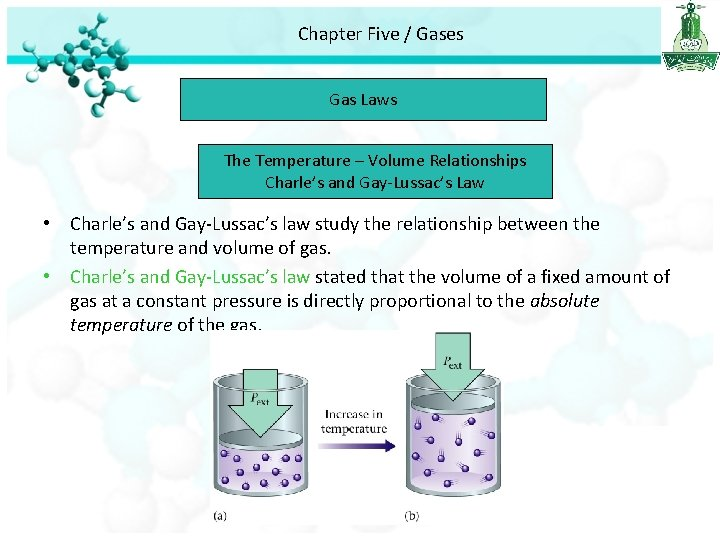Chapter Five / Gases Gas Laws The Temperature – Volume Relationships Charle’s and Gay-Lussac’s Law • Charle’s and Gay-Lussac’s law study the relationship between the temperature and volume of gas. • Charle’s and Gay-Lussac’s law stated that the volume of a fixed amount of gas at a constant pressure is directly proportional to the absolute temperature of the gas.Chapter Five / Gases Gas Laws- Charle’s Law TαV Charle’s Law T in Kelvin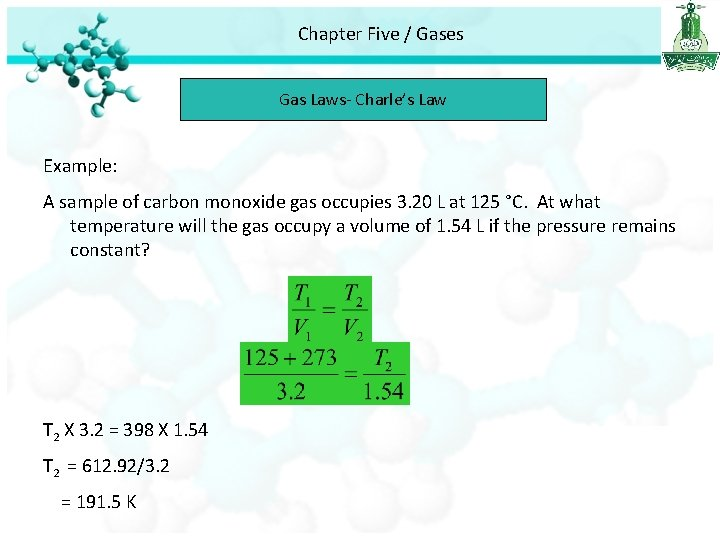Chapter Five / Gases Gas Laws- Charle’s Law Example: A sample of carbon monoxide gas occupies 3. 20 L at 125 °C. At what temperature will the gas occupy a volume of 1. 54 L if the pressure remains constant? T 2 X 3. 2 = 398 X 1. 54 T 2 = 612. 92/3. 2 = 191. 5 K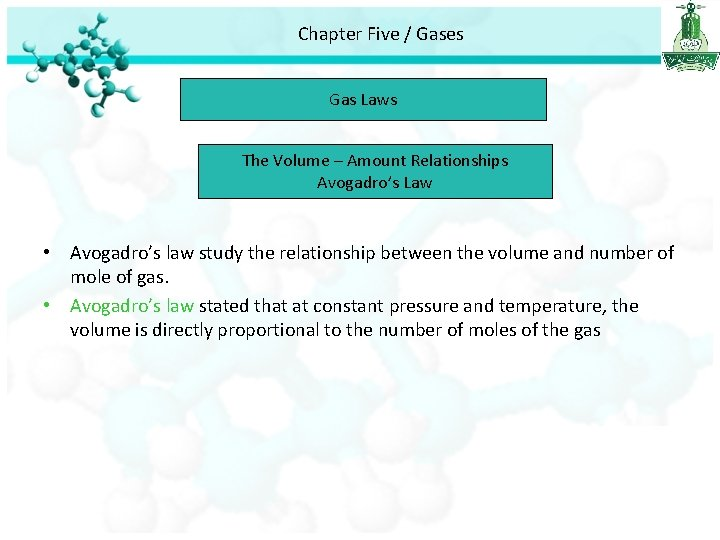Chapter Five / Gases Gas Laws The Volume – Amount Relationships Avogadro’s Law • Avogadro’s law study the relationship between the volume and number of mole of gas. • Avogadro’s law stated that at constant pressure and temperature, the volume is directly proportional to the number of moles of the gasChapter Five / Gases Gas Laws- Avogadro’s Law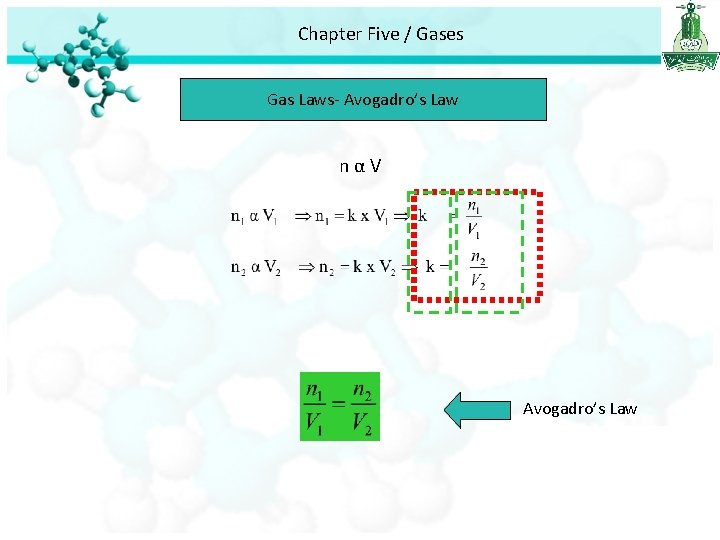Chapter Five / Gases Summery of Gas Laws P 1 V 1 = P 2 V 2 Boyle’s Law Constant T and n Charle’s Law Constant P and n Avogadro’s Law Constant P and TChapter Five / Gases Ideal Gas Equation We know that PV=n. RT Ideal Gas Equation R Gas constant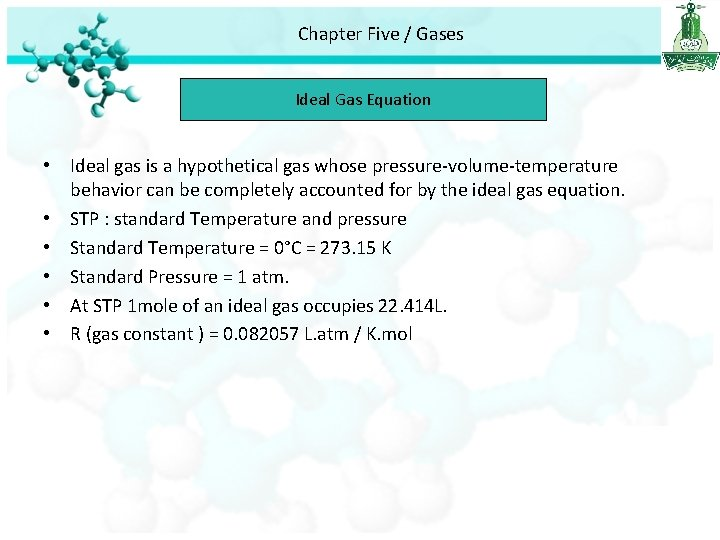Chapter Five / Gases Ideal Gas Equation • Ideal gas is a hypothetical gas whose pressure-volume-temperature behavior can be completely accounted for by the ideal gas equation. • STP : standard Temperature and pressure • Standard Temperature = 0°C = 273. 15 K • Standard Pressure = 1 atm. • At STP 1 mole of an ideal gas occupies 22. 414 L. • R (gas constant ) = 0. 082057 L. atm / K. mol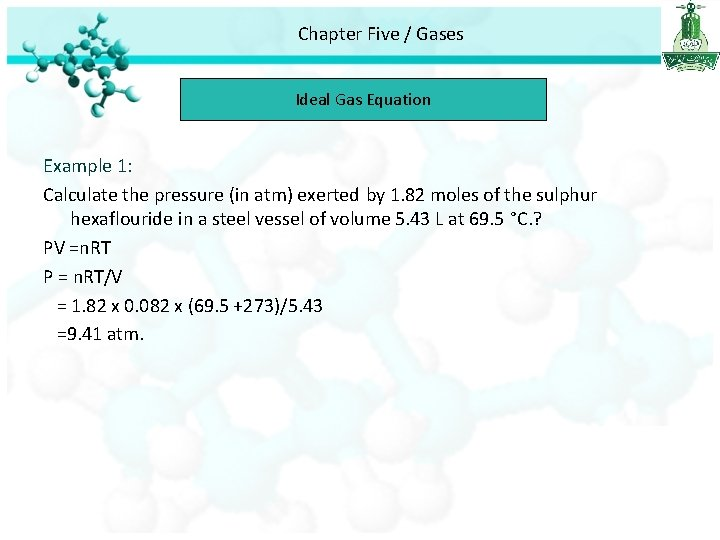Chapter Five / Gases Ideal Gas Equation Example 1: Calculate the pressure (in atm) exerted by 1. 82 moles of the sulphur hexaflouride in a steel vessel of volume 5. 43 L at 69. 5 °C. ? PV =n. RT P = n. RT/V = 1. 82 x 0. 082 x (69. 5 +273)/5. 43 =9. 41 atm.Chapter Five / Gases Ideal Gas Equation Example 2: Calculate the volume (in liters) occupied by 7. 40 g of NH 3 at STP condition. ? PV=n. RT V = n. RT/P n = mass/molar mass n = 7. 40 / 17 = 0. 435 mol V= 0. 435 X 0. 082 X 273 / 1 = 9. 74 L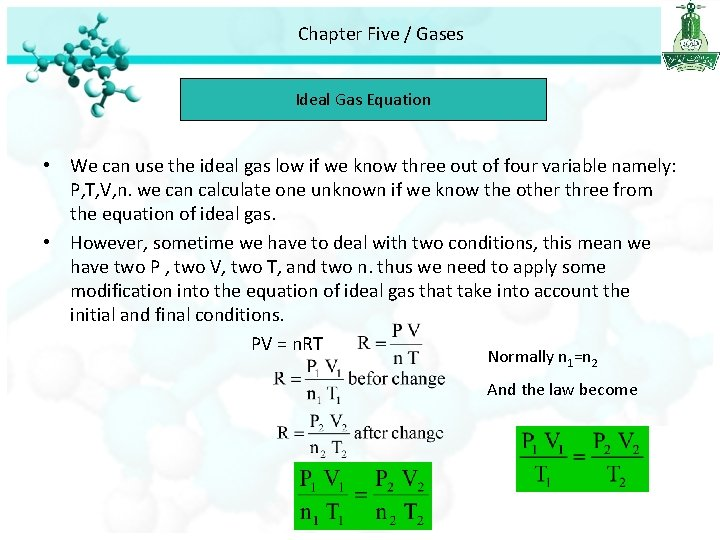Chapter Five / Gases Ideal Gas Equation • We can use the ideal gas low if we know three out of four variable namely: P, T, V, n. we can calculate one unknown if we know the other three from the equation of ideal gas. • However, sometime we have to deal with two conditions, this mean we have two P , two V, two T, and two n. thus we need to apply some modification into the equation of ideal gas that take into account the initial and final conditions. PV = n. RT Normally n 1=n 2 And the law become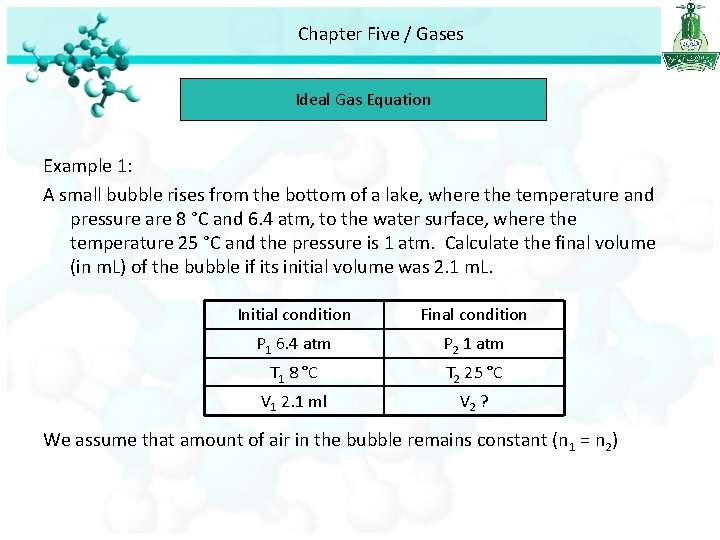Chapter Five / Gases Ideal Gas Equation Example 1: A small bubble rises from the bottom of a lake, where the temperature and pressure are 8 °C and 6. 4 atm, to the water surface, where the temperature 25 °C and the pressure is 1 atm. Calculate the final volume (in m. L) of the bubble if its initial volume was 2. 1 m. L. Initial condition Final condition P 1 6. 4 atm P 2 1 atm T 1 8 °C T 2 25 °C V 1 2. 1 ml V 2 ? We assume that amount of air in the bubble remains constant (n 1 = n 2)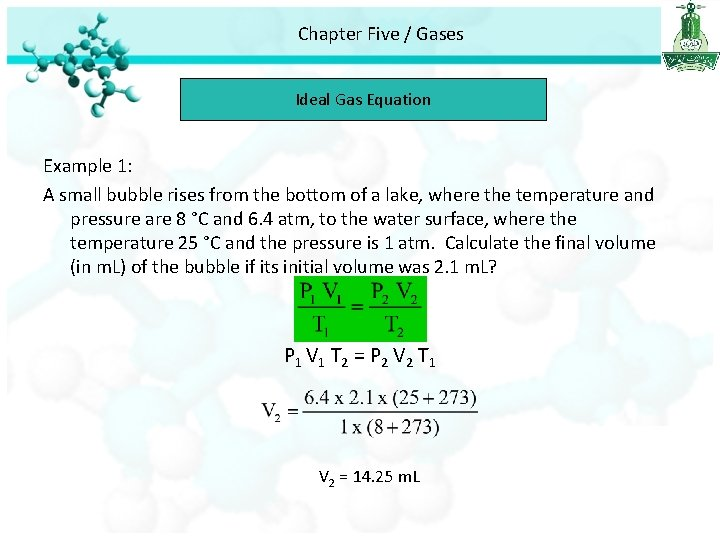Chapter Five / Gases Ideal Gas Equation Example 1: A small bubble rises from the bottom of a lake, where the temperature and pressure are 8 °C and 6. 4 atm, to the water surface, where the temperature 25 °C and the pressure is 1 atm. Calculate the final volume (in m. L) of the bubble if its initial volume was 2. 1 m. L? P 1 V 1 T 2 = P 2 V 2 T 1 V 2 = 14. 25 m. LChapter Five / Gases Ideal Gas Equation Example 2: An inflated helium balloon with a volume of 0. 55 L at sea level (1 atm) is allowed to rise to a high of 6. 5 km. where the pressure is about 0. 40 atm. Assuming that the temperature remains constant. What is the final volume of the balloon? We assume that n 1 = n 2 and T 1 = T 2 P 1 V 1 = P 2 V 2 1 x 0. 55 = 0. 4 x V 2 = 0. 55 / 0. 4 = 1. 4 L